GFG App
Open AppBrowser
Continue

A Cartesian plane is defined as the intersection of two perpendicular number lines namely the x-axis (horizontal) and the y-axis (vertical). Using these axes, one can describe any point in the plane using an ordered pair of numbers. The position of any point on the Cartesian plane is represented by using two numbers: (x, y)

• The first number, x, is the horizontal position of the point from the origin. It is called the x-coordinate.
• The second number, y, is the vertical position of the point from the origin. It is called the y-coordinate.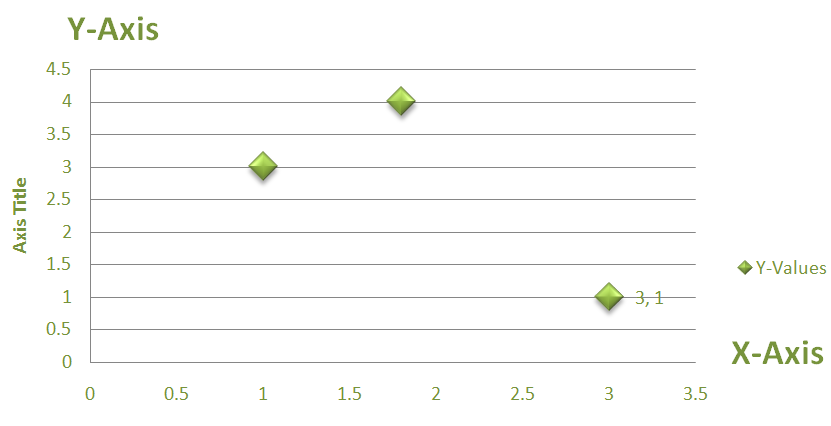### How to plot a value in a cartesian plane?

First, check the value to which axis does it belongs to?  Whether it is X-axis or Y-axis. After Conforming to the axis now you need to know the value given is a positive value or negative value? Then it is easy to find in which quadrant it is to be placed.

• Quadrants I(+ve, +ve): Here both x, y values are positive i.e (x > 0, y > 0)
• Quadrants II(-ve, +ve): Here x is negative value and y is positive value i.e (x < 0, y > 0)
• Quadrants III(-ve, -ve): Here both x, y values are negative i.e (x < 0, y < 0)
• Quadrants IV(+ve, -ve): Here  x is positive value and y is negative value i.e (x > 0, y < 0)
• Origin: The value where both the X-axis and Y-axis values are 0. This can be represented as O (0, 0) i.e Origin(0, 0).

### Examples

After considering the value in X-axis and Y-axis plot them into the plane

i) 2, 3

Solution:

Plot 2,3 in the plane where (2 > 0, 3 > 0). Thus they are in 1st quadrant. The plot is as the following: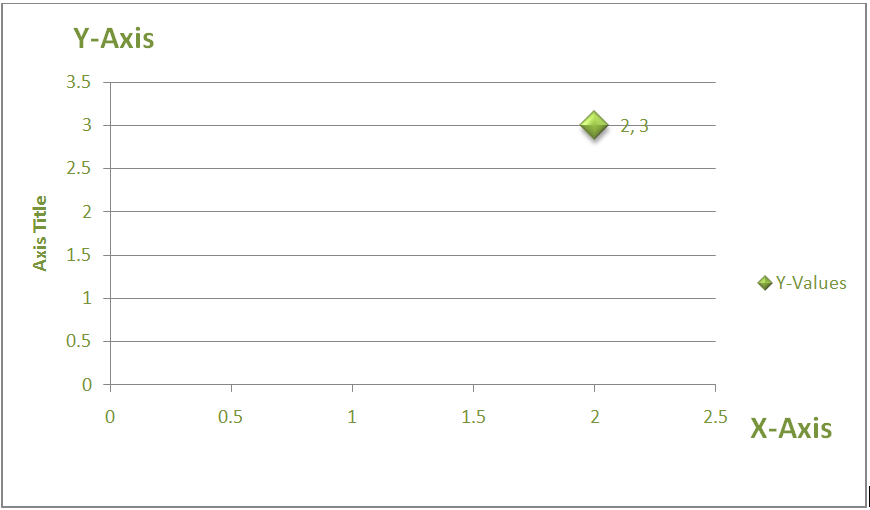ii) 2, -3

Solution:

Plot  2, -3 in the plane where (2 > 0, -3 < 0). Thus They are placed in the 4th quadrant. The plot is as the following: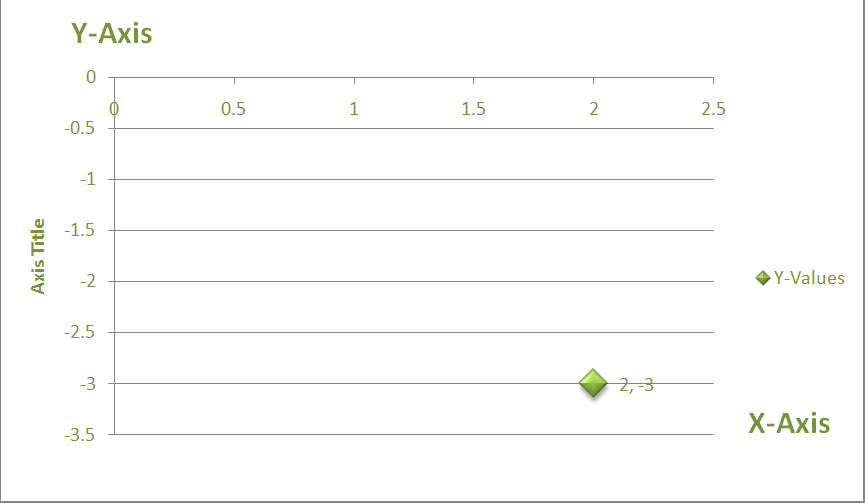iii) -2, -3

Solution:

Plot 2, 3 in the plane where (-2 < 0, -3 < 0). Thus they are in 3rd quadrant. The plot is as the following: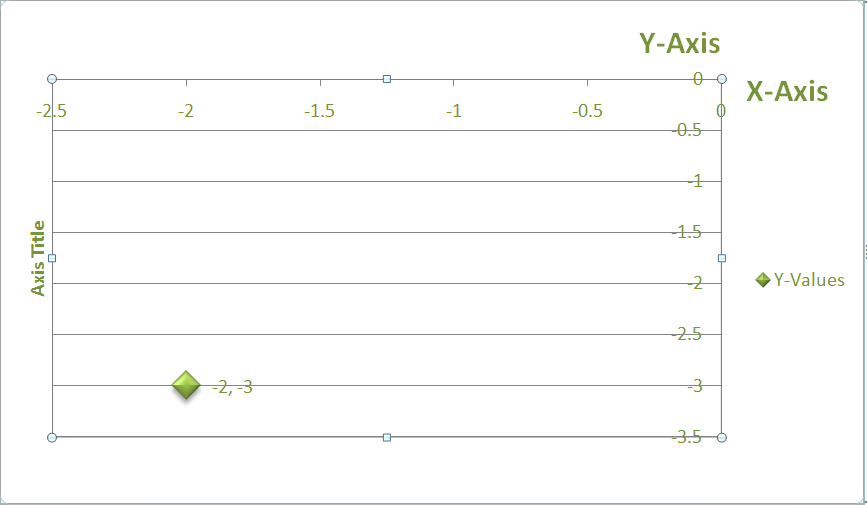iv) -2, 3

Solution:

Plot -2, 3 in the plane where (-2 < 0, 3 > 0). Thus they are in the 2nd quadrant. The plot is as the following: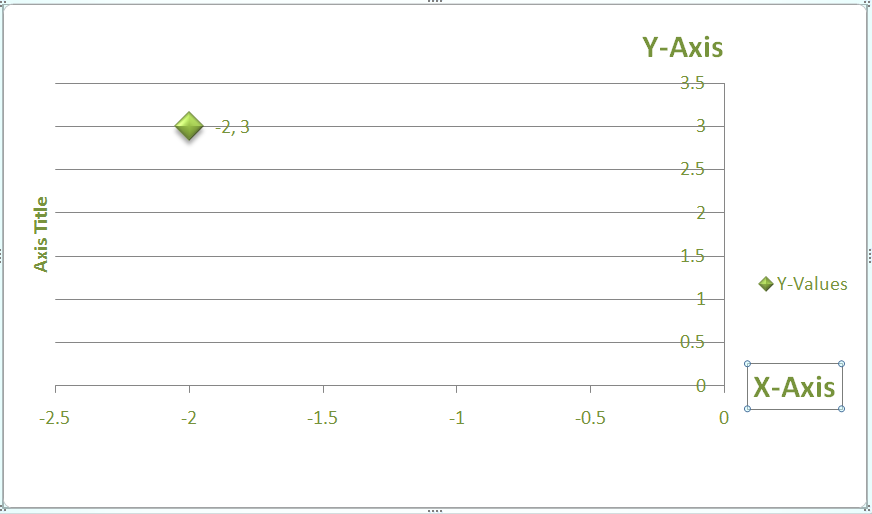v) 4, -4

Solution:

Plot 4, -4 in the plane where (4 > 0, -4 < 0). Thus they are in the 4th quadrant. The plot is as the following: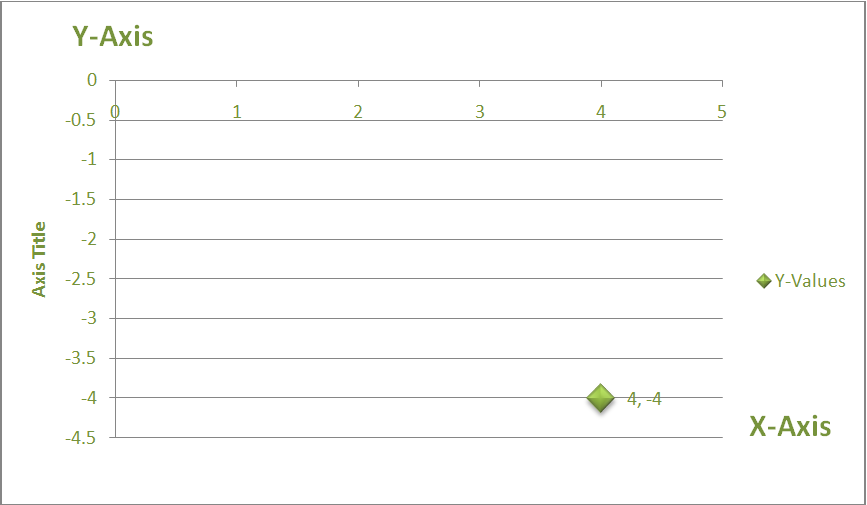My Personal Notes arrow_drop_up